# Angles of Special Measures:

There are some elegant and accurate methods to construct some angles of special sizes which do not require the use of the protractor.

## 1. Constructing a 60° angle:

Step 1: Draw a line l and mark a point O on it.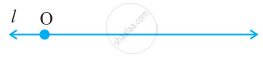Step 2: Place the pointer of the compasses at O and draw an arc of convenient radius which cuts the line bar"PQ" at a point say, A.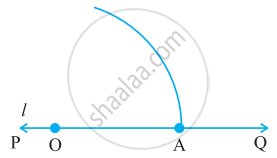Step 3: With the pointer at A (as centre), now draw an arc that passes through O.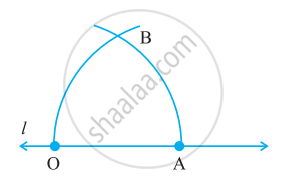Step 4: Let the two arcs intersect at B. Join OB. We get ∠BOA whose measure is 60°.

## 2. Constructing a 30° angle:

To construct an angle of 30°, we need to draw an angle of 60° as above then bisect it with the process of an angle bisector.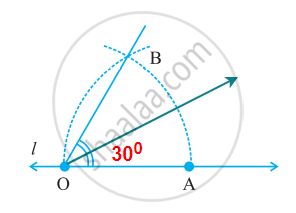## 3. Constructing a 90° angle:

Step 1: Draw a line l and mark a point P on it. Now taking P as a centre and with a convenient radius, draw an arc of a circle which intersects line l at Q.

Step 2: Taking Q as a centre and with the same radius as before, draw an arc intersecting the previously drawn arc at R.

Step 3: Taking R as a centre and with the same radius as before, draw an arc intersecting the arc at S.

Step 4: Taking R and S as a centre, draw an arc of the same radius to intersect each other at T.

Step 5: Join PT, which is the required ray making 90° with line l.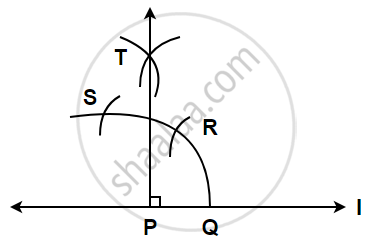## 4. Constructing a 45° angle:

Draw an angle of 90° then bisect it to make an angle of 45°.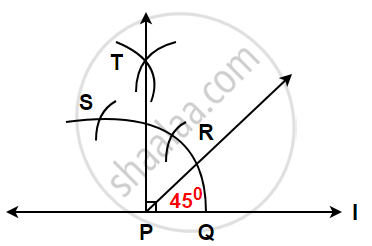## 5. Constructing a 120° angle:

An angle of 120° is nothing but twice of an angle of 60°.

Therefore, it can be constructed as follows :

Step 1: Draw any line PQ and take a point O on it.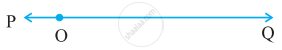Step 2: Place the pointer of the compasses at O and draw an arc of convenient
radius which cuts the line at A.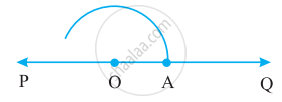Step 3: Without disturbing the radius on the compasses, draw an arc with A as the centre which cuts the first arc at B.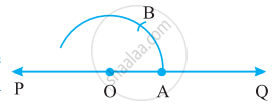Step 4: Again without disturbing the radius on the compasses and with B as centre, draw an arc which cuts the first arc at C.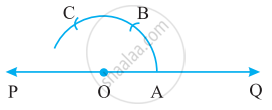Step 5: Join OC, ∠COA is the required angle whose measure is 120°.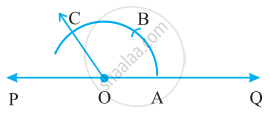If you would like to contribute notes or other learning material, please submit them using the button below.

### Shaalaa.com

Angles of Special Measures [00:05:02]
S
0%